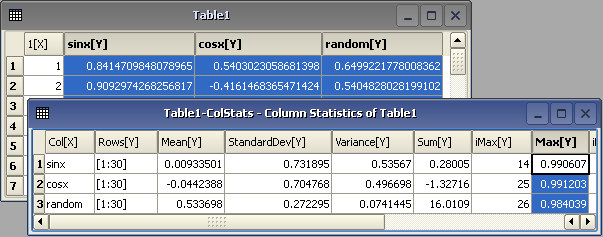# The Statistics Menu

The commands in this menu are only available if a table window is selected.

## Descriptive Statistics

### Statistics on Columns

The Statistics -> Descriptive Statistics -> Statistics on Columns command creates a new table providing basic statistical information about the selected columns in the active table: average, variance, standard deviation, max value, etc...The quantities to be computed and displayed in the columns of the result table can be customized via the Descriptive Statistics tab of the Preferences dialog.If you select several columns in one table, one line of statistical information will be created for each selected column. However, you can not select columns from different tables and obtain one single table of statistics.

It is possible to edit in place the names of the columns and the range of rows to be analysed by double-clicking the corresponging cells. However, you can not set different row ranges for different columns. A valid range of rows must be entered in the Rows column, under the form [startRow:endRow], whith startRow lower than endRow. Entering the value -1 for the endRow means the whole column.

### Statistics on Rows

The Statistics -> Descriptive Statistics -> Statistics on Rows command creates a new table providing basic statistical information about the selected rows in the active table: average, variance, standard deviation, max value, etc. Statistics are calculated only on numeric data in visible columns. Usually, statistics should be calculated on data of a similar type - Y values for example. This feature permits selection of only those columns that should be included in the statistical calculation.

It is possible to edit in place the index of the rows to be analysed by double-clicking the corresponging cells.

### Frequency Count

The Statistics -> Descriptive Statistics -> Frequency Count command calculates the frequency distribution of the data in the selected column. The Frequency Count dialog is popped up which allows selecting the data range and the bin width. If more than one column is selected, an error dialog will pop-up.

### Normality Test (Shapiro-Wilk)...

The Statistics -> Descriptive Statistics -> Normality Test (Shapiro-Wilk)... command computes a Shapiro-Wilk test on the selected column to determine how well the column conforms to a normal distribution. This command opens the Normality Test (Shapiro-Wilk) dialog.

## Hypothesis-Testing

### One Sample t-Test...

The Statistics -> Hypothesis Testing -> One Sample t-Test... command performs a Student's t-test on the selected column of data to estimate the statistical likelihood that the mean has a specific value. This command opens the One sample t-Test dialog.

### Two Sample t-Test...

The Statistics -> Hypothesis Testing -> Two Sample t-Test... command performs a Student's t-test on two selected columns of data to estimate the statistical likelihood that the means are the same. This command opens the Two sample t-Test dialog.

### Chi-square Test for Variance...

The Statistics -> Hypothesis Testing -> Chi-square Test for Variance... command performs a Chi-square test on the selected column of data to estimate the statistical likelihood that the variance has a specific value. This command opens the Chi-square Test dialog.

## ANOVA

### One-Way ANOVA...

The Statistics -> ANOVA -> One-Way ANOVA... command performs a one-way analysis of variance on the selected columns of data. This command opens the One-Way ANOVA dialog.

### Two-Way ANOVA...

The Statistics -> ANOVA -> Two-Way ANOVA... command performs a Two-way analysis of variance on the selected columns of data. This command opens the Two-Way ANOVA dialog.

## Nonparametric Tests

### One Sample Wilcoxon Signed Rank Test...

The Statistics -> Nonparametric Tests -> One Sample Wilcoxon Signed Rank Test... command can be used in order to determine whether the median of a data sample is equal to a specific value. This command opens the One Sample Wilcoxon Signed Rank Test dialog.

### Paired Sample Sign Test...

The Statistics -> Nonparametric Tests -> Paired Sample Sign Test... command can be used in order to test for consistent differences between pairs of observations. This command opens the Sign Test dialog.

### Paired Sample Wilcoxon Signed Rank Test...

The Statistics -> Nonparametric Tests -> Paired Sample Wilcoxon Signed Rank Test... command can be used in order to determine whether two dependent samples were selected from populations having the same distribution. This command opens the Paired Sample Wilcoxon Signed Rank Test dialog.

### Kolmogorov-Smirnov Test...

The Statistics -> Nonparametric Tests -> Kolmogorov-Smirnov Test... command can be used in order to estimate the statistical likelihood that two samples are drawn from the same distribution. This command opens the Two Sample Kolmogorov-Smirnov Test dialog.

### Mann-Whitney Test...

The Statistics -> Nonparametric Tests -> Mann-Whitney Test... command can be used in order to determine whether two independent samples were selected from populations having the same distribution. This command opens the Mann-Whitney Test dialog.

### Kruskal-Wallis ANOVA...

The Statistics -> Nonparametric Tests -> Kruskal-Wallis ANOVA... is a method for testing whether several samples originate from the same distribution. This command opens the Kruskal-Wallis ANOVA dialog.

### Mood's Median Test...

The Statistics -> Nonparametric Tests -> Mood's Median Test... is a method for testing whether several samples originate from the same distribution. This command opens the Mood's median test dialog.

### Friedman ANOVA...

The Statistics -> Nonparametric Tests -> Friedman ANOVA... is a method for testing whether several samples originate from the same distribution. This command opens the Friedman ANOVA dialog.